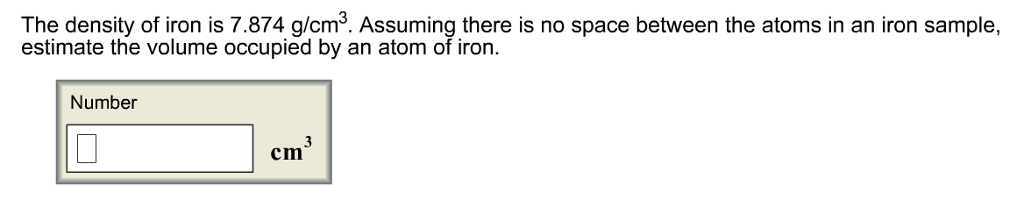# Problem: The density of iron is 7.874 g/cm^3. Assuming there is no space between the atoms in an iron sample, estimate the volume occupied by an atom of iron.

###### FREE Expert Solution
95% (428 ratings)###### Problem Details

The density of iron is 7.874 g/cm^3. Assuming there is no space between the atoms in an iron sample, estimate the volume occupied by an atom of iron.